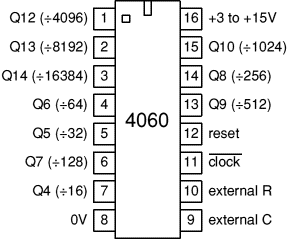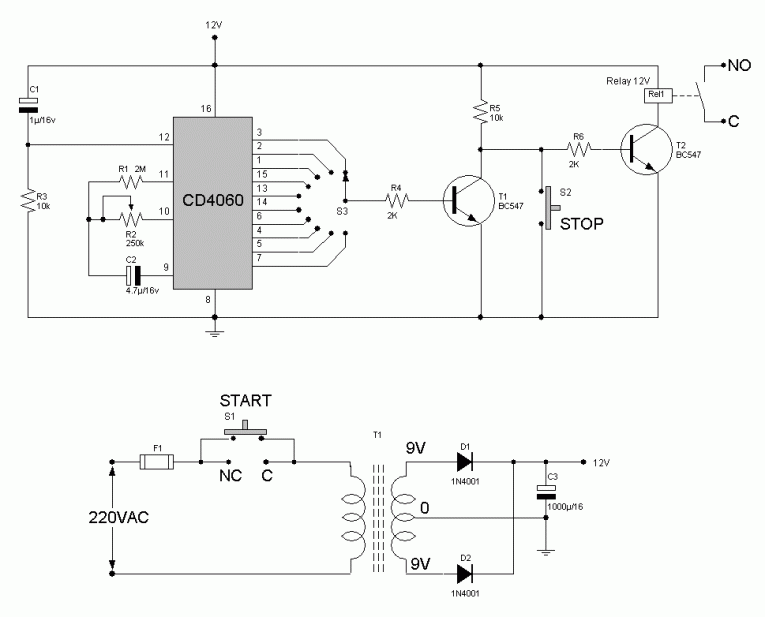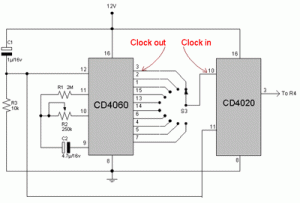# Timer with 4060

This is an electronics timer by CMOS CD4060 which is CMOS 14 Stage Ripple-Carry Binary Counter/Divider and Oscillator.Output Qn is the nth stage of the counter, representing 2n, for example Q4 is 24 = 16 (1/16 of clock frequency) and Q14 is 214 = 16384 (1/16384 of clock frequency).
Note that Q1-3 and Q11 are not available.T = 2.2 x R2 x C2 ———(1)
If this circuits need max interval time is 5 hours 20 minute or 320 minute.So the period of oscillator determine by
320 x 60 /8192 =2.34 Second
When 60 use to scale to second and 8192 = 213

From (1) we can find the R2 by fix C2 to the standard value 4.7uF.

R2 = 2.34 /(2.2 x 4.7uF) = 226 K

choose R2 = 220K
and from data sheet R1 is 2R2 to 10R2 so we choose 2.2M

When S3 selected the timer have the time as the following table

 S3 connect to pin Time 7 19 Second 5 38 Second 4 75 Second 6 2.5 Minute 14 5 Minute 13 10 Minute 15 20 Minute 1 80 Minute 2 160 Minte (2 hours 40 minute) 3 320 Minute (5 hours 20 minute)

If you need more time you can cascade with CD4020 by connect the output of the above circuit to input of CD4020 as show in the buttom picture.